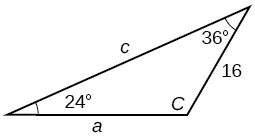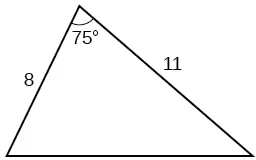Precalculus

# Review Exercises

PrecalculusReview Exercises

### Review Exercises

##### Non-right Triangles: Law of Sines

For the following exercises, assume $α α$ is opposite side $a,β a,β$ is opposite side $b, b,$ and $γ γ$ is opposite side $c. c.$ Solve each triangle, if possible. Round each answer to the nearest tenth.

1.

$β=50°,a=105,b=45 β=50°,a=105,b=45$

2.

$α=43.1°,a=184.2,b=242.8 α=43.1°,a=184.2,b=242.8$

3.

Solve the triangle.4.

Find the area of the triangle.5.

A pilot is flying over a straight highway. He determines the angles of depression to two mileposts, 2.1 km apart, to be 25° and 49°, as shown in Figure 1. Find the distance of the plane from point $A A$ and the elevation of the plane.

Figure 1
##### Non-right Triangles: Law of Cosines
6.

Solve the triangle, rounding to the nearest tenth, assuming $α α$ is opposite side $a,β a,β$ is opposite side $b, b,$ and $γ γ$ s opposite side $c:a=4,b=6,c=8. c:a=4,b=6,c=8.$

7.

Solve the triangle in Figure 2, rounding to the nearest tenth.

Figure 2
8.

Find the area of a triangle with sides of length 8.3, 6.6, and 9.1.

9.

To find the distance between two cities, a satellite calculates the distances and angle shown in Figure 3 (not to scale). Find the distance between the cities. Round answers to the nearest tenth.

Figure 3
##### Polar Coordinates
10.

Plot the point with polar coordinates $( 3, π 6 ). ( 3, π 6 ).$

11.

Plot the point with polar coordinates $( 5,− 2π 3 ) ( 5,− 2π 3 )$

12.

Convert $( 6,− 3π 4 ) ( 6,− 3π 4 )$ to rectangular coordinates.

13.

Convert $( −2, 3π 2 ) ( −2, 3π 2 )$ to rectangular coordinates.

14.

Convert $( 7,−2 ) ( 7,−2 )$ to polar coordinates.

15.

Convert $( −9,−4 ) ( −9,−4 )$ to polar coordinates.

For the following exercises, convert the given Cartesian equation to a polar equation.

16.

$x=−2 x=−2$

17.

$x 2 + y 2 =64 x 2 + y 2 =64$

18.

$x 2 + y 2 =−2y x 2 + y 2 =−2y$

For the following exercises, convert the given polar equation to a Cartesian equation.

19.

$r=7cosθ r=7cosθ$

20.

$r= −2 4cosθ+sinθ r= −2 4cosθ+sinθ$

For the following exercises, convert to rectangular form and graph.

21.

$θ= 3π 4 θ= 3π 4$

22.

$r=5secθ r=5secθ$

##### Polar Coordinates: Graphs

For the following exercises, test each equation for symmetry.

23.

$r=4+4sinθ r=4+4sinθ$

24.

$r=7 r=7$

25.

Sketch a graph of the polar equation $r=1−5sinθ. r=1−5sinθ.$ Label the axis intercepts.

26.

Sketch a graph of the polar equation $r=5sin( 7θ ). r=5sin( 7θ ).$

27.

Sketch a graph of the polar equation $r=3−3cosθ r=3−3cosθ$

##### Polar Form of Complex Numbers

For the following exercises, find the absolute value of each complex number.

28.

$−2+6i −2+6i$

29.

$4−​3i 4−​3i$

Write the complex number in polar form.

30.

$5+9i 5+9i$

31.

$1 2 − 3 2 ​i 1 2 − 3 2 ​i$

For the following exercises, convert the complex number from polar to rectangular form.

32.

$z=5cis( 5π 6 ) z=5cis( 5π 6 )$

33.

$z=3cis( 40° ) z=3cis( 40° )$

For the following exercises, find the product $z 1 z 2 z 1 z 2$ in polar form.

34.

$z 1 =2cis( 89° ) z 1 =2cis( 89° )$

$z 2 =5cis( 23° ) z 2 =5cis( 23° )$

35.

$z 1 =10cis( π 6 ) z 1 =10cis( π 6 )$

$z 2 =6cis( π 3 ) z 2 =6cis( π 3 )$

For the following exercises, find the quotient $z 1 z 2 z 1 z 2$ in polar form.

36.

$z 1 =12cis( 55° ) z 1 =12cis( 55° )$

$z 2 =3cis( 18° ) z 2 =3cis( 18° )$

37.

$z 1 =27cis( 5π 3 ) z 1 =27cis( 5π 3 )$

$z 2 =9cis( π 3 ) z 2 =9cis( π 3 )$

For the following exercises, find the powers of each complex number in polar form.

38.

Find $z 4 z 4$ when $z=2cis( 70° ) z=2cis( 70° )$

39.

Find $z 2 z 2$ when $z=5cis( 3π 4 ) z=5cis( 3π 4 )$

For the following exercises, evaluate each root.

40.

Evaluate the cube root of $z z$ when $z=64cis( 210° ). z=64cis( 210° ).$

41.

Evaluate the square root of $z z$ when $z=25cis( 3π 2 ). z=25cis( 3π 2 ).$

For the following exercises, plot the complex number in the complex plane.

42.

$6−2i 6−2i$

43.

$−1+3i −1+3i$

##### Parametric Equations

For the following exercises, eliminate the parameter $t t$ to rewrite the parametric equation as a Cartesian equation.

44.

${ x( t )=3t−1 y( t )= t { x( t )=3t−1 y( t )= t$

45.

${ x(t)=−cost y(t)=2 sin 2 t { x(t)=−cost y(t)=2 sin 2 t$

46.

Parameterize (write a parametric equation for) each Cartesian equation by using $x( t )=acost x( t )=acost$ and $y(t)=bsint y(t)=bsint$ for $x 2 25 + y 2 16 =1. x 2 25 + y 2 16 =1.$

47.

Parameterize the line from $(−2,3) (−2,3)$ to $(4,7) (4,7)$ so that the line is at $(−2,3) (−2,3)$ at $t=0 t=0$ and $(4,7) (4,7)$ at $t=1. t=1.$

##### Parametric Equations: Graphs

For the following exercises, make a table of values for each set of parametric equations, graph the equations, and include an orientation; then write the Cartesian equation.

48.

${ x( t )=3 t 2 y( t )=2t−1 { x( t )=3 t 2 y( t )=2t−1$

49.

${ x(t)= e t y(t)=−2 e 5t { x(t)= e t y(t)=−2 e 5t$

50.

${ x(t)=3cost y(t)=2sint { x(t)=3cost y(t)=2sint$

51.

A ball is launched with an initial velocity of 80 feet per second at an angle of 40° to the horizontal. The ball is released at a height of 4 feet above the ground.

1. Find the parametric equations to model the path of the ball.
2. Where is the ball after 3 seconds?
3. How long is the ball in the air?
##### Vectors

For the following exercises, determine whether the two vectors, $u u$ and $v, v,$ are equal, where $u u$ has an initial point $P 1 P 1$ and a terminal point $P 2 , P 2 ,$ and $v v$ has an initial point $P 3 P 3$ and a terminal point $P 4 . P 4 .$

52.

$P 1 =( −1,4 ), P 2 =( 3,1 ), P 3 =( 5,5 ) P 1 =( −1,4 ), P 2 =( 3,1 ), P 3 =( 5,5 )$ and $P 4 =( 9,2 ) P 4 =( 9,2 )$

53.

$P 1 =( 6,11 ), P 2 =( −2,8 ), P 3 =( 0,−1 ) P 1 =( 6,11 ), P 2 =( −2,8 ), P 3 =( 0,−1 )$ and $P 4 =( −8,2 ) P 4 =( −8,2 )$

For the following exercises, use the vectors $u=2i−j,v=4i−3j, u=2i−j,v=4i−3j,$ and $w=−2i+5j w=−2i+5j$ to evaluate the expression.

54.

uv

55.

2vu + w

For the following exercises, find a unit vector in the same direction as the given vector.

56.

a = 8i − 6j

57.

b = −3ij

For the following exercises, find the magnitude and direction of the vector.

58.

$〈 6,−2 〉 〈 6,−2 〉$

59.

$〈 −3,−3 〉 〈 −3,−3 〉$

For the following exercises, calculate $u⋅v. u⋅v.$

60.

u = −2i + j and v = 3i + 7j

61.

u = i + 4j and v = 4i + 3j

62.

Given v $=〈 −3,4 〉 =〈 −3,4 〉$ draw v, 2v, and $1 2 1 2$ v.

63.

Given the vectors shown in Figure 4, sketch u + v, uv and 3v.

Figure 4
64.

Given initial point $P 1 =( 3,2 ) P 1 =( 3,2 )$ and terminal point $P 2 =( −5,−1 ), P 2 =( −5,−1 ),$ write the vector $v v$ in terms of $i i$ and $j. j.$ Draw the points and the vector on the graph.

Order a print copy

As an Amazon Associate we earn from qualifying purchases.